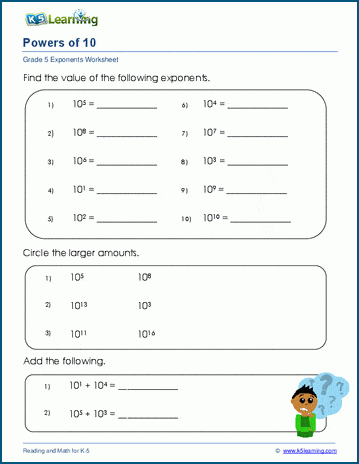# Exponents Worksheets for Grade 5 Students

In our grade 5 math section, we introduce exponents to students. These worksheets help students practice reading and writing exponents, including the special case of powers of ten.

## What is an exponent?

An exponent refers to the number of times a number is multiplied by itself. For example, “2 to the power of 3”, written like 23 means:

2 x 2 x 2 = 8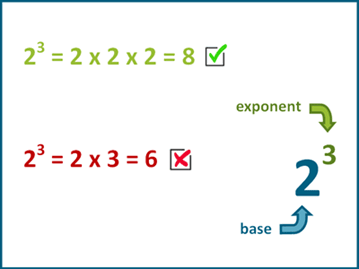Our grade 5 exponents worksheets introduce the use of exponents.

## Introducing exponents worksheets

Students learn about exponents as repeated multiplication of a base number in these worksheets.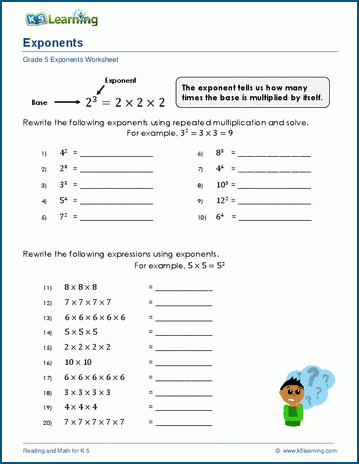Once they’ve covered the introductory worksheets, students move onto working with exponents.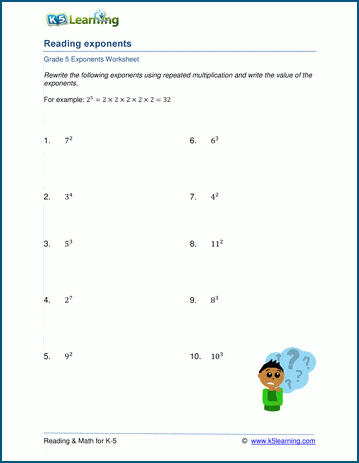## Students write exponents

These worksheets ask students to rewrite expressions as exponents.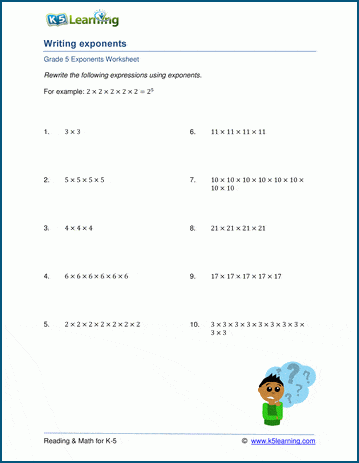## Powers of ten worksheets

Finally, we have a set of worksheets where students review reading and writing powers of ten.